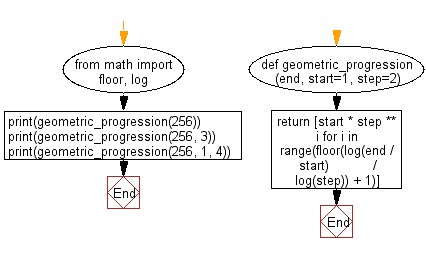﻿ Python: Geometric progression - w3resource# Python: Geometric progression

## Python List: Exercise - 244 with Solution

Write a Python program to initialize a list containing the numbers in the specified range where start and end are inclusive and the ratio between two terms is step. Returns an error if step equals 1.

• Use range(), math.log() and math.floor() and a list comprehension to create a list of the appropriate length, applying the step for each element.
• Omit the second argument, start, to use a default value of 1.
• Omit the third argument, step, to use a default value of 2.

Sample Solution:

Python Code:

``````from math import floor, log
def geometric_progression(end, start=1, step=2):
return [start * step ** i for i in range(floor(log(end / start)
/ log(step)) + 1)]
print(geometric_progression(256))
print(geometric_progression(256, 3))
print(geometric_progression(256, 1, 4))
```
```

Sample Output:

```[1, 2, 4, 8, 16, 32, 64, 128, 256]
[3, 6, 12, 24, 48, 96, 192]
[1, 4, 16, 64, 256]
```

Flowchart:## Visualize Python code execution:

The following tool visualize what the computer is doing step-by-step as it executes the said program:

Python Code Editor:

Have another way to solve this solution? Contribute your code (and comments) through Disqus.

What is the difficulty level of this exercise?

Test your Python skills with w3resource's quiz

﻿

## Python: Tips of the Day

```print(2_000_000)
```2000000